NCERT Solutions Chapter 10 - Circles (I), Class 9, Maths

# NCERT Solutions Chapter 10 - Circles (I), Class 9, Maths - Extra Documents & Tests for Class 9

Exercise 10.1

1. Fill in the blanks:
(i) The centre of a circle lies in ____________ of the circle. (exterior/ interior)
(ii) A point, whose distance from the centre of a circle is greater than its radius lies in __________ of the circle. (exterior/ interior)
(iii) The longest chord of a circle is a _____________ of the circle.
(iv) An arc is a ___________ when its ends are the ends of a diameter.
(v) Segment of a circle is the region between an arc and _____________ of the circle.
(vi) A circle divides the plane, on which it lies, in _____________ parts.

(i)   The centre of a circle lies in interior of the circle. (exterior/interior)
(ii)  A point, whose distance from the centre of a circle is greater than its radius lies in exterior of the circle. (exterior/interior)
(iii) The longest chord of a circle is a diameter of the circle.
(iv) An arc is a semicircle when its ends are the ends of a diameter.
(v) Segment of a circle is the region between an arc and chord of the circle.
(vi) A circle divides the plane, on which it lies, in three parts.

(i) Line segment joining the centre to any point on the circle is a radius of the circle.
(ii) A circle has only finite number of equal chords.
(iii) If a circle is divided into three equal arcs, each is a major arc.
(iv) A chord of a circle, which is twice as long as its radius, is a diameter of the circle.
(v) Sector is the region between the chord and its corresponding arc.
(vi) A circle is a plane figure.

(i) True.
All the line segment from the centre to the circle is of equal length.
(ii) False.
We can draw infinite numbers of equal chords.
(iii) False.
We get major and minor arcs for unequal arcs. So, for equal arcs on circle we can't say it is major arc or minor arc.
(iv) True.
A chord which is twice as long as radius must pass through the centre of the circle and is diameter to the circle.
(v) False.
Sector is the region between the arc and the two radii of the circle.
(vi) True.
A circle can be drawn on the plane.

Exercise 10.2

1. Recall that two circles are congruent if they have the same radii. Prove that equal chords of congruent circles subtend equal angles at their centres.

A circle is a collection of points whose every every point is equidistant from the centre. Thus, two circles can only be congruent when they the distance of every point of the both circle is equal from the centre.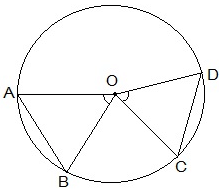Given,
AB = CD (Equal chords)
To prove,
∠AOB = ∠COD
Proof,
In ΔAOB and ΔCOD,
AB = CD (Given)
∴ ΔAOB ≅ ΔCOD (SSS congruence condition)
Thus, ∠AOB = ∠COD by CPCT.
Equal chords of congruent circles subtend equal angles at their centres.

2. Prove that if chords of congruent circles subtend equal angles at their centres, then the chords are equal.Given,
∠AOB = ∠COD (Equal angles)
To prove,
AB = CD
Proof,
In ΔAOB and ΔCOD,
∠AOB = ∠COD (Given)
∴ ΔAOB ≅ ΔCOD (SAS congruence condition)
Thus, AB = CD by CPCT.
If chords of congruent circles subtend equal angles at their centres, then the chords are equal.

Exercise 10.3

1. Draw different pairs of circles. How many points does each pair have in common? What is the maximum number of common points?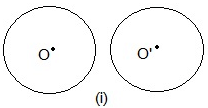No point is common.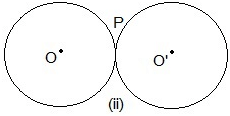One point P is common.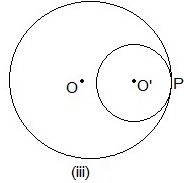One point P is common.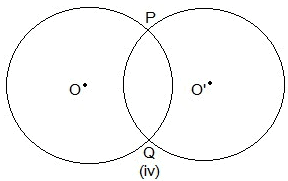Two points P and Q are common.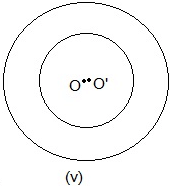No point is common.

2. Suppose you are given a circle. Give a construction to find its centre.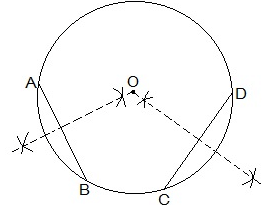Steps of construction:
Step I: A circle is drawn.
Step II: Two chords AB and CD are drawn.
Step III: Perpendicular bisector of the chords AB and CD are drawn.
Step IV: Let these two perpendicular bisector meet at a point. The point of intersection of these two perpendicular bisector is the centre of the circle.

3. If two circles intersect at two points, prove that their centres lie on the perpendicular bisector of the common chord.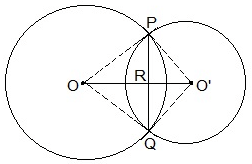Given,
Two circles which intersect each other at P and Q.
To prove,
OO' is perpendicular bisector of PQ.
Proof,
In ΔPOO' and ΔQOO',
OO' = OO' (Common)
∴ ΔPOO' ≅ ΔQOO' (SSS congruence condition)
Thus,
∠POO' = ∠QOO' --- (i)
In ΔPOR and ΔQOR,
∠POR = ∠QOR (from i)
OR = OR (Common)
∴ ΔPOR ≅ ΔQOR (SAS congruence condition)
Thus,
∠PRO = ∠QRO
also,
∠PRO + ∠QRO = 180°
⇒ ∠PRO = ∠QRO = 180°/2 = 90°
Hence,
OO' is perpendicular bisector of PQ.

Exercise 10.4

1. Two circles of radii 5 cm and 3 cm intersect at two points and the distance between their centres is 4 cm. Find the length of the common chord.

OP = 5cm, PS = 3cm and OS = 4cm.
also, PQ = 2PR
Let RS be x.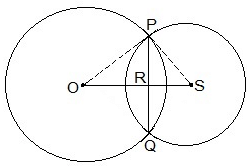In ΔPOR,
OP= OR+ PR2
⇒ 5= (4-x)+ PR2
⇒ 25 = 16 + x- 8x +  PR2
⇒ PR2 = 9 - x2 + 8x --- (i)

In ΔPRS,
PS= PR+ RS2
⇒ 3= PR2 + x2
⇒  PR2 = 9 - x2 --- (ii)

Equating (i) and (ii),
9 - x2 + 8x = 9 - x2
⇒ 8x = 0
⇒ x = 0
Putting the value of x in (i) we get,
PR2 = 9 - 02
⇒ PR = 3cm
Length of the cord PQ = 2PR = 2×3 = 6cm

2. If two equal chords of a circle intersect within the circle, prove that the segments of one chord are equal to corresponding segments of the other chord.

Given,
AB and CD are chords intersecting at E.
AB = CD
To prove,
AE = DE and CE = BE
Construction,
OM ⊥ AB and ON ⊥ CD. OE is joined.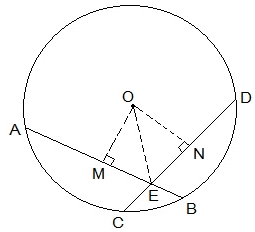Proof,
OM bisects AB (OM ⊥ AB)
ON bisects CD (ON ⊥ CD)
As AB = CD thus,
AM = ND --- (i)
and MB = CN --- (ii)
In ΔOME and ΔONE,
∠OME = ∠ONE (Perpendiculars)
OE = OE (Common)
OM = ON (AB = CD and thus equidistant from the centre)
ΔOME ≅ ΔONE by RHS congruence condition.
ME = EN by CPCT --- (iii)
From (i) and (ii) we get,
AM + ME = ND + EN
⇒ AE = ED
From (ii)  and (iii) we get,
MB - ME = CN - EN
⇒ EB = CE

3. If two equal chords of a circle intersect within the circle, prove that the line joining the point of intersection to the centre makes equal angles with the chords.

Given,
AB and CD are chords intersecting at E.
AB = CD, PQ is the diameter.
To prove,
∠BEQ = ∠CEQ
Construction,
OM ⊥ AB and ON ⊥ CD. OE is joined.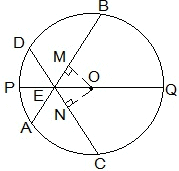In ΔOEM and ΔOEN,
OM = ON (Equal chords are equidistant from the centre)
OE = OE (Common)
∠OME = ∠ONE (Perpendicular)
ΔOEM ≅ ΔOEN by RHS congruence condition.
Thus,
∠MEO = ∠NEO by CPCT
⇒ ∠BEQ = ∠CEQ

4. If a line intersects two concentric circles (circles with the same centre) with centre O at A, B, C and D, prove that AB = CD (see Fig. 10.25).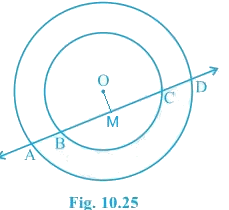OM ⊥ AD is drawn from O.
⇒ AM = MD --- (i)
also, OM bisects BC as OM ⊥ BC.
⇒ BM = MC --- (ii)
From (i) and (ii),
AM - BM = MD - MC
⇒ AB = CD

5. Three girls Reshma, Salma and Mandip are playing a game by standing on a circle of radius 5m drawn in a park. Reshma throws a ball to Salma, Salma to Mandip, Mandip to Reshma. If the distance between Reshma and Salma and between Salma and Mandip is 6m each, what is the distance between Reshma and Mandip?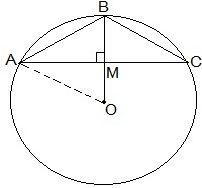Let A, B and C represent the positions of Reshma, Salma and Mandip respectively.
AB = 6cm and BC = 6cm.
BM ⊥ AC is drawn.
ABC is an isosceles triangle as AB = BC, M is mid-point of AC. BM is perpendicular bisector of AC and thus it passes through the centre of the circle.
Let AM = y and OM = x then BM = (5-x).
Applying Pythagoras theorem in ΔOAM,
OA= OM+ AM2
⇒ 5= x+ y--- (i)
Applying Pythagoras theorem in ΔAMB,
AB= BM+ AM2
⇒ 6= (5-x)+ y2 --- (ii)
Subtracting (i) from (ii), we get
36 - 25 = (5-x)- x2 -
⇒ 11 = 25 - 10x
⇒ 10x = 14 ⇒ x= 7/5
Substituting the value of x in (i), we get
y+ 49/25 = 25
⇒ y2 = 25 - 49/25
⇒ y2 = (625 - 49)/25
⇒ y2 = 576/25
⇒ y = 24/5
Thus,
AC = 2×AM = 2×y = 2×(24/5) m = 48/5 m = 9.6 m
Distance between Reshma and Mandip is 9.6 m.

6. A circular park of radius 20m is situated in a colony. Three boys Ankur, Syed and David are sitting at equal distance on its boundary each having a toy telephone in his hands to talk each other. Find the length of the string of each phone.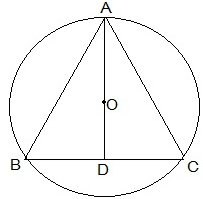Let A, B and C represent the positions of Ankur, Syed and David respectively. All three boys at equal distances thus ABC is an equilateral triangle.
AD ⊥ BC is drawn. Now, AD is median of ΔABC and it passes through the centre O.
Also, O is the centroid of the ΔABC. OA is the radius of the triangle.
Let the side of a triangle a metres then BD = a/2 m.
Applying Pythagoras theorem in ΔABD,
OA = 2/3 AD ⇒ 20 m = 2/3 × √3a/2
⇒ a = 20√3 m
Length of the string is 20√3 m.

The document NCERT Solutions Chapter 10 - Circles (I), Class 9, Maths | Extra Documents & Tests for Class 9 is a part of the Class 9 Course Extra Documents & Tests for Class 9.
All you need of Class 9 at this link: Class 9

## Extra Documents & Tests for Class 9

1 videos|228 docs|21 tests

## FAQs on NCERT Solutions Chapter 10 - Circles (I), Class 9, Maths - Extra Documents & Tests for Class 9

 1. What are the different parts of a circle?Ans. A circle has several parts: - Center: The point in the middle of the circle from which all points on the circle are equidistant. - Radius: The distance from the center of the circle to any point on the circle. - Diameter: The distance across the circle passing through the center, which is twice the length of the radius. - Chord: A line segment that connects two points on the circle. - Arc: A portion of the circumference of the circle. - Sector: The region enclosed by two radii and the corresponding arc.
 2. How can we find the circumference of a circle?Ans. The circumference of a circle can be found using the formula C = 2πr, where C represents the circumference and r represents the radius of the circle. If the diameter of the circle is given, we can use the formula C = πd, where d represents the diameter.
 3. What is the relationship between the radius and diameter of a circle?Ans. The diameter of a circle is always twice the length of its radius. In other words, if we know the radius of a circle, we can find the diameter by multiplying the radius by 2. Conversely, if we know the diameter, we can find the radius by dividing the diameter by 2.
 4. How can we find the area of a circle?Ans. The area of a circle can be found using the formula A = πr^2, where A represents the area and r represents the radius of the circle. If the diameter of the circle is given, we can use the formula A = π(d/2)^2, where d represents the diameter.
 5. How can we find the length of an arc in a circle?Ans. The length of an arc in a circle can be found using the formula L = (θ/360) × 2πr, where L represents the length of the arc, θ represents the central angle in degrees, and r represents the radius of the circle. If the central angle is given in radians, we can use the formula L = θr, where L represents the length of the arc, θ represents the central angle in radians, and r represents the radius of the circle.

## Extra Documents & Tests for Class 9

1 videos|228 docs|21 testsExplore Courses for Class 9 examSignup to see your scores go up within 7 days! Learn & Practice with 1000+ FREE Notes, Videos & Tests.
10M+ students study on EduRev
Track your progress, build streaks, highlight & save important lessons and more!
Related Searches

,

,

,

,

,

,

,

,

,

,

,

,

,

,

,

,

,

,

,

,

,

,

,

,

,

,

,

;